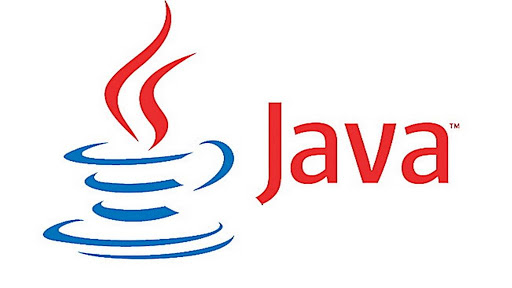### Basics of Java Programming - Part 1

####Datatypes in Java

• int 4 bytes
• short int 2 bytes
• long int 8 bytes
• byte 1 byte
• float  4 bytes
• double 8 bytes
• char 2 bytes

#### Character to ASCII conversion in JAVA

class CharToASCII {
public static void main(String a[]) {
char c1 = 'A';
char c2 = 'a';
System.out.print((int)c1); // OUTPUT: 65
System.out.print((int)c2); // OUTPUT: 97
System.out.print((char)66); // OUTPUT: B  --> ASCII to int conversion
}
}

#### "printf" is also available in JAVA

class PrintfInJava {
public static void main(String a[]) {
int i = 4;
int j = 7;
int i+j;
System.out.printf("Addition of %d and %d is %d", i, j, k); // OUTPUT: 65
}
}

#### Binary Literals

public class BinaryLiterals {
public static void main(String a[]) {
// i, j and k are same representation
int i = 0b10000000;
int j = 0B10000000;
int k = 0b100_00_000;
System.out.printf("value of i is %d, j is %d and k is %d", ijk);
}
}
//OUTPUT: value of i is 128, j is 128 and k is 128

#### Post increment

public class PostIncrement {
public static void main(String a[]) {
int i = 10;
i = i++;
/*
i = i++; is similar to:
int temp;
temp = i;
i++;
i = temp;
//OUTPUT 10
*/
System.out.println("value of i is " + i );
int j = 7;
int k;
k = j++;
System.out.println("\n value of k is: "+ k + " and j is: "+ );
}
}

//OUTPUT:
// value of i is 10
//  value of k is: 7 and j is: 8

#### Bitwise left and right shift operator

public class LeftAndRightShiftOps {
public static void main(String a[]) {
int i = 25; // Binary  1 1 0 0 1
int j = i << 2; // Binary 1 1 0 0 1 0 0
int k = i >> 2; // Binary 1 1 0
System.out.printf("value of j is %d \nvalue of k is %d", j, k);
}
}

//OUTPUT:
// value of j is 100
// value of k is 6